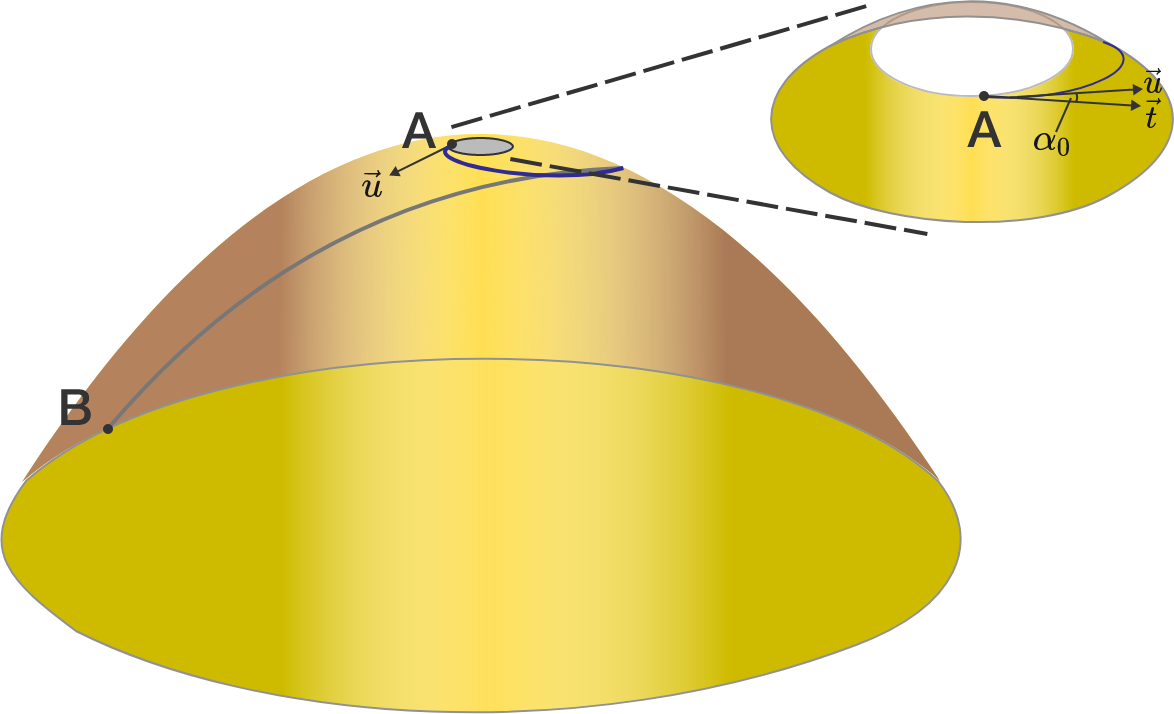# Volcano-watching

The surface of a volcano is represented by the frustum of the paraboloid $z = 225 - x^2 - y^2$ for $0 \le z \le 224.$ A geological research station is located at point $A=(1,0,224)$ on the edge of the crater of the volcano. The main research center for the volcano is located at point $B=(15,0,0).$

The scientists studying the volcano have established a path from $A$ to $B$ that passes all the way around the volcano exactly once—so that measurements can be taken of all sides of the volcano—and has the shortest possible length just in case the volcano shows signs of erupting.

What is the angle $\alpha_0$ that the tangent vector $\mathbf{t}$ to the path makes with the horizontal tangent vector $\mathbf{u}$ to the surface of the volcano at point $A?$ Give your answer in degrees, correct to $1$ decimal place.

Note: This integral will (in)definitely help.×